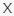September 23, 2023
Latest:
Bloomerspace

# A Math Quiz You Can Easily Crack Answers

Q 1. 227.5 + 42.5 = ?

Choices:
290
280
270
233

270

Q 2. 54 ÷ 3 x 1 = ?

Choices:
17
18
19
16

18

Q 3. 54 is 75% of what number

Choices:
90
72
84
80

72

Q 4. Which of the following numbers is divisible by only itself?

Choices:
27
33
23
32

23

Q 5. What is the number in the upper half of a fraction called?

Choices:
Denominator
Componendo
Numerator
Ratio

Numerator

Q 6. Which one of the following is twice the sum of their individual digits?

Choices:
24
18
40
27

18

Q 7. 9+9+7 is the same as?

Choices:
6.5 x 5
11 x 2.5
12 x 2.5
12.5 x 2

12.5 x 2

Q 8. What is the symbol of omega?

Choices:

π
ρ
α

Q 9. In which place is the value of “5” in 0.2859?

Choices:
Tenth’s
Thousandth’s
Ten Thousandth’s
Hundredth’s

Thousandth’s

Q 10. How many milliliters are there in a 1 liter?

Choices:
1,000
0.001
0.01
10,000